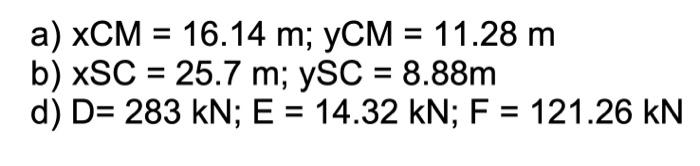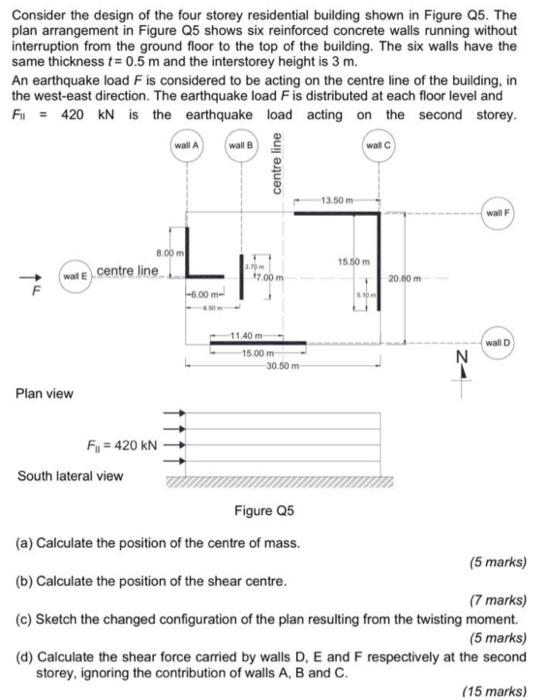Question!Thank you
a) $$x C M=16.14 \mathrm{~m} ; y C M=11.28 \mathrm{~m}$$ b) $$x S C=25.7 \mathrm{~m} ; y S C=8.88 \mathrm{~m}$$ d) $$D=283 \mathrm{kN} ; E=14.32 \mathrm{kN} ; F=121.26 \mathrm{kN}$$ Consider the design of the four storey residential building shown in Figure Q5. The plan arrangement in Figure Q5 shows six reinforced concrete walls running without interruption from the ground floor to the top of the building. The six walls have the same thickness $$t=0.5 \mathrm{~m}$$ and the interstorey height is $$3 \mathrm{~m}$$. An earthquake load $$F$$ is considered to be acting on the centre line of the building, in the west-east direction. The earthquake load $$F$$ is distributed at each floor level and E. $$=490 \mathrm{kN}$$ ie the sarthnuake Inad antinn on the carnne etaraw South la Figure Q5 (a) Calculate the position of the centre of mass. (b) Calculate the position of the shear centre. (5 marks) (7 marks) (c) Sketch the changed configuration of the plan resulting from the twisting moment. (5 marks) (d) Calculate the shear force carried by walls $$D, E$$ and $$F$$ respectively at the second storey, ignoring the contribution of walls A, B and C. (15 marks)

We have an Answer from Expert